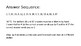# Rational Exponents Stations ReviewSubject
Resource Type
File Type

Presentation (Powerpoint) File

(674 KB)
Product Rating
Standards
• Product Description
• StandardsNEW

This activity will give students a chance to test their understanding of:
1. Converting between radical form and exponential form

2. Simplifying expressions involving radical exponents

3. Evaluating expressions involving radical exponents

4. Solving exponential equations in the form a^x = b

It is engaging, makes it easy for you to check student understanding, and has minimal set up/ prep time.

Use square root and cube root symbols to represent solutions to equations of the form 𝘹² = 𝘱 and 𝘹³ = 𝘱, where 𝘱 is a positive rational number. Evaluate square roots of small perfect squares and cube roots of small perfect cubes. Know that √2 is irrational.
Know and apply the properties of integer exponents to generate equivalent numerical expressions. For example, 3² × (3⁻⁵) = (3⁻³) = 1/3³ = 1/27.
Total Pages
N/A
Included
Teaching Duration
40 minutes
Report this Resource to TpT
Reported resources will be reviewed by our team. Report this resource to let us know if this resource violates TpT’s content guidelines.